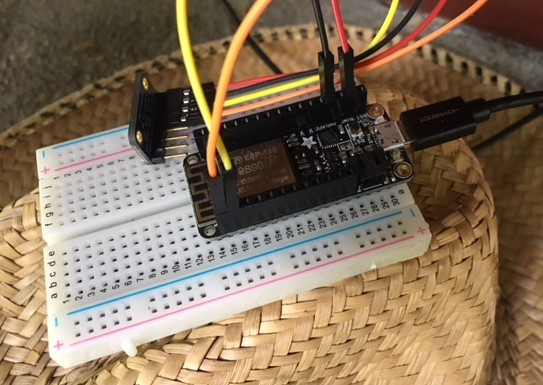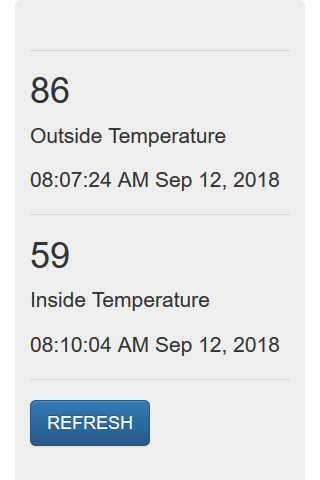## Statistics in Python using the statistics module

In this post, we'll look at a couple of statistics functions in Python. These statistics functions are part of the Python Standard Library in the statistics module. The four functions we'll use in this post are common in statistics:

• mean - average value
• median - middle value
• mode - most often value
• standard …

## Solving Two Equations for Two Unknowns and a Statics Problem with SymPy and Python

SymPy (http://www.sympy.org) is a Python library for symbolic math.

In symbolic math, symbols represent mathematical expressions. In a numerical calculation, the value of pi is stored as an estimate of pi, a floating point number close to 3.14.... In a symbolic math expression, the value of …

## Solving Equations and Writing Expressions with SymPy and Python

SymPy (http://www.sympy.org) is a Python library for symbolic math.

In symbolic math, symbols are used to represent mathematical expressions. An example of a symbolic math expression is below:

$$x^{2} + y^{2} = z$$

In the expression above, we have the variables $x$, $y$ and $z$.

If we …

## Building an IoT Server with flask and Python - Part 6 - upload code to ESP8266-based WiFi weather stations

Date Series Part 6 of Building an IoT Server with Flask and Python Tags python / flask / IoT / sensorThis is the sixth part of a series of posts about building an Internet of Things (IoT) server with flask, Python and ESP8266 microcontrollers. In this post, we'll add some code to our ESP8266-based weather stations. The code we upload to the ESP8266 microcontrollers programs the WiFi weather stations to …

## Building an IoT Server with flask and Python - Part 5 Adding a DatabaseThis is the fifth part of a series of posts about building an Internet of Things (IoT) server with flask, Python and ESP8266 microcontrollers. In this post, we'll add a sqlite3 database to our flask IoT server to store all the temperature data points that come in from our ESP8266-based …